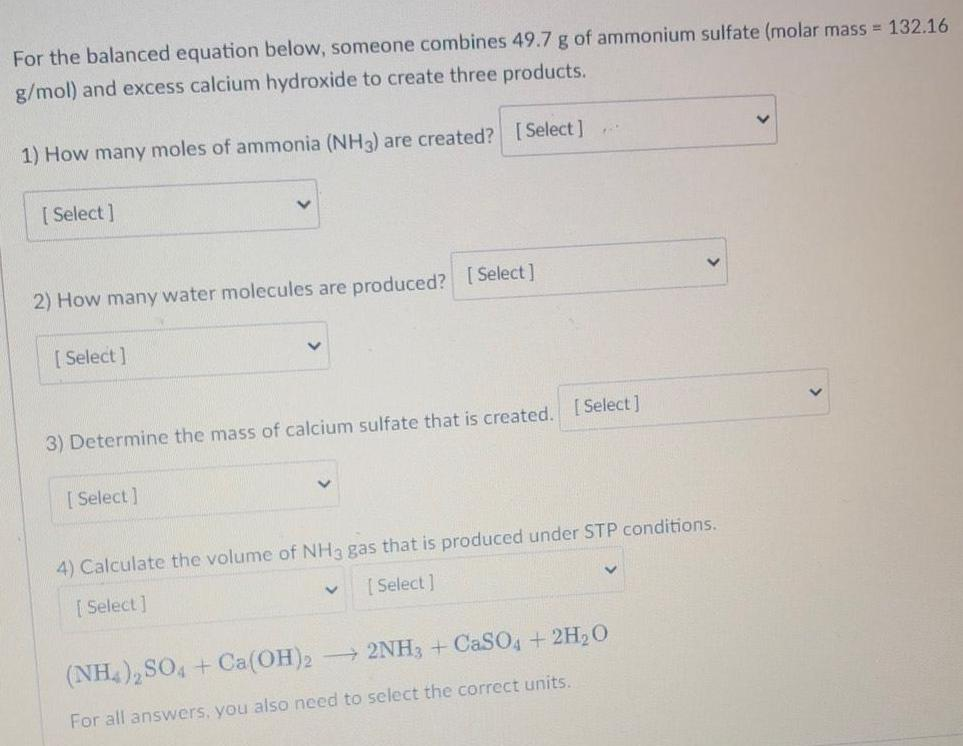Question:

# For the balanced equation below, someone combines 49.7 g of

Last updated: 7/10/2022For the balanced equation below, someone combines 49.7 g of ammonium sulfate (molar mass = 132.16 g/mol) and excess calcium hydroxide to create three products. 1) How many moles of ammonia (NH3) are created? 2) How many water molecules are produced? 3) Determine the mass of calcium sulfate that is created. 4) Calculate the volume of NH3 gas that is produced under STP conditions. (NH4)2SO4 + Ca(OH)2 → 2NH3 + CaSO4 + 2H2O For all answers, you also need to select the correct units.